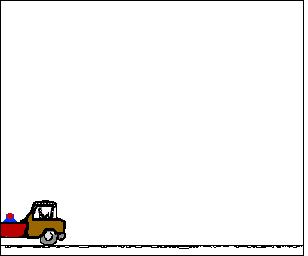## The Truck and The Ball

Imagine a pickup truck moving with a constant speed along a city street. In the course of its motion, a ball is projected straight upwards by a launcher located in the bed of the truck. Imagine as well that the ball does not encounter a significant amount of airresistance. What will be the path of the ball and where will it be located with respect to the pickup truck? How can the motion of the ball be described? And where will the ball land with respect to the truck? The animation below depicts such a situation. The path of the ball and the truck are shown; additionally, the velocity components (horizontal and vertical) are represented by arrows in the animation.As can be seen from the above animation, the ball follows a parabolic path and remains directly above the launcher at all times. As the ball rises towards its peak, it undergoes a downward acceleration. An upwardly moving ball which is slowing down is said to be undergoing a downward acceleration. As the ball falls, it also undergoes a downward acceleration. A downwardly moving ball which is gaining speed is said to have a downward acceleration. This downward acceleration is attributed to the downward force of gravity which acts upon the ball. If the ball's motion can be approximated as projectile motion (that is, if the influence of air resistance can be assumed negligible), then there will be no horizontal acceleration. In the absence of horizontal forces, there would be a constant velocity in the horizontal direction. This explains why the ball would be located directly above the launcher from which it is projected.

Many would insist that there is a horizontal force acting upon the ball since it has a horizontal motion. This is simply not the case. The horizontal motion of the ball is the result of its own inertia. When projected from the truck, the ball already possessed a horizontal motion, and thus will maintain this state of horizontal motion unless acted upon by a horizontal force. An object in motion will continue in motion with the same speed and in the same direction ... (Newton's first law). Remind yourself continuously: forces do not cause motion; rather, forces cause accelerations.

For more information on physical descriptions of motion, visit The Physics Classroom Tutorial. Detailed information is available there on the following topics:

Acceleration of Gravity

Direction of Acceleration

Projectiles

Characteristics of a Projectile's Trajectory

Newton's First Law of Motion

Inertia and the State of Motion